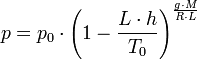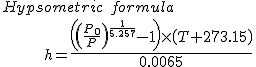# Calculating air pressure from altitude, RH and temperature?

## Homework Statement

[/B]
This is a practical problem, no homework question.

I'd like to determine pressure (and O2 partial pressure) at altitude, yet following a more realistic real-world calc including measured data like relative humidity (RH) and temperature at that altitude, yet all I can find are more generic formulas accounting sealevel temperature and standard temperature lapse rate, which seem a less accurate approach given that I'd have to guess sealevel temperature while measuring RH and temperature at a certain height is direct rather than computed data.

## Homework Equations

Closest I can find is:where

• sea level standard atmospheric pressure p0 = 101325 Pa
• sea level standard temperature T0 = 288.15 K
• Earth-surface gravitational acceleration g = 9.80665 m/s2.
• temperature lapse rate L = 0.0065 K/m
• universal gas constant R = 8.31447 J/(mol·K)
• molar mass of dry air M = 0.0289644 kg/mol
or reversing this one:## The Attempt at a Solution

• However both seem rather inaccurate to me since they assume dry air which luckily never occurs in realworld (moist air is surely less dense) and I can easily measure both RH and temperature at my altitude (and/or calculate dew point) rather than estimate them from sealevel temp and lapse rate.

I've found some online calculator/forms however I'm not skilled enough to read the sourcecode and get the equations from there.

Does the formula I'm looking for exist or it has to be put together from aviation books I'm not aware of?
Thank you

Allison

Chestermiller
Mentor
The humidity of the air has very little effect on the vertical pressure profile. That's why it's typically not included. What do you think the mole fraction of water vapor is in the air at ground level with a 50 % humidity at 25 C? How much does this affect the average molecular weight of the air?

I see thank you, maybe I'm mistaken with air density lowered by humidity. However, why is there no measured T rather than resorting to sealevel T and lapse rate?

Chestermiller
Mentor
I see thank you, maybe I'm mistaken with air density lowered by humidity. However, why is there no measured T rather than resorting to sealevel T and lapse rate?
Vertical temperature profiles are routinely measured by meteorologists on a daily and even hourly basis. The sea level T and lapse rate just provide average values over time for rough calculations.